Park City Mathematics Institute 2009 Course Notes Bowen Kerins and Darryl Yong

These course materials are licensed under a Creative Commons Attribution-NonCommercial-ShareAlike 4.0 International License.Some Questions and Problems in Arithmetic: This course will investigate questions like these:

• In how many ways can an integer be written as the sum of two squares?
• In how many ways can an integer be written as the sum of four squares?
• What's the probability that an integer picked at random has no perfect square factor?
• What's the probability that two integers picked at random have no common factor?
• Which linear functions f(x) = ax + b (a and b integers) generate infinitely many prime numbers for integer values of x?
• What is the probability that an integer picked at random between 1 and 1020 is a prime number?

The real goal of the course is to answer the following question:

How are all of the above questions related?

In this three week course, you will investigate questions like those above and develop underlying structural similarities among them. In particular, you will see how to use the algebra of sequences and series as a general- purpose tool for these investigations. No prior knowledge of number theory or sequences and series is assumed, and by the end of three weeks, you'll be astounded at what you've discovered about arithmetic and its applications to the 7-12 curriculum.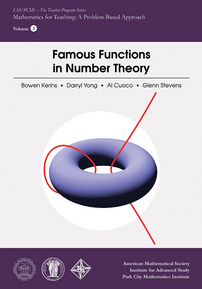Now availableFamous Functions in Number Theory published by American Mathematical Society
 June 29 - July 3, 2009 Download Week 1 [PDF file]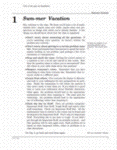July 6 - 10, 2009 Download Week 2 [PDF file]July 13 - 17, 2009 Download Week 3 [PDF file]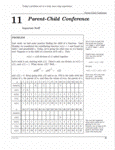NOTE: As you select any of the links listed above, they will download as PDF files. To open PDF files use Adobe Acrobat Reader, available free from Adobe: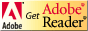Unless otherwise indicated all of these materials are licensed under a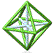© 2001 - 2019 Park City Mathematics Institute IAS/Park City Mathematics Institute is an outreach program of the Institute for Advanced Study, 1 Einstein Drive, Princeton, NJ 08540. Send questions or comments to: Suzanne Alejandre